[TOC]

# Overview

What is observability ?

In control theory, observability is a measure for how well internal states of a system can be inferred by knowledge of its external outputs.

What is consistency ?

A recursive estimator is consistent when the estimation errors are zero-mean and have covariance matrix equal to that reported by the estimator.

Observability $$\longrightarrow$$ Consistency

Mismatch (actual vs true) in observability $$\longrightarrow$$ Inconsistency

VINS observability properties $$\longrightarrow$$ estimator inconsistency

# Basics

## Nullspace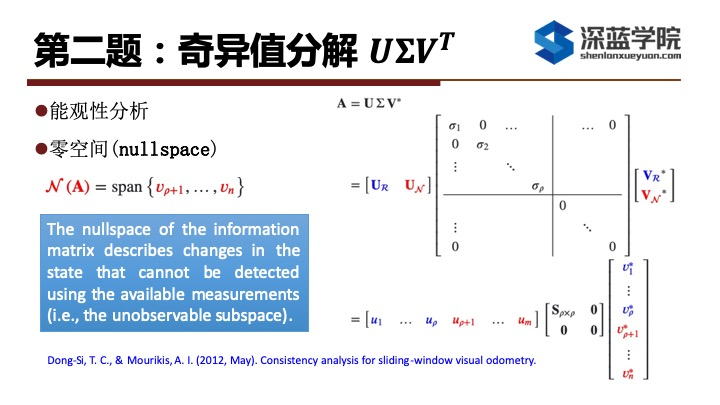## Lie Derivative 

\begin{aligned} h(x):& \; R^n \rightarrow R \\ f(x):& \; R^n \rightarrow R^n \\ g(x):& \; R^n \rightarrow R^n \end{aligned}

$L_f h = \nabla h \cdot f = \left( \frac{\partial h}{\partial x} \right)^T f$

$$L_g L_f h$$ 结果依然是个标量

$L_g L_f h = L_g (L_f h) = \nabla(L_f h) g = \left( \frac{\partial (L_f h)}{\partial x} \right)^T g = \left( \frac{\partial \left( (\frac{\partial h}{\partial x})^T f \right)}{\partial x} \right)^T g$

# Observability Analysis 

• what: 控制理论中的 可观察性（observability） 是指系统可以由其外部输出推断其内部状态的程度。

• why: 为了能让系统不可观的维度与真实系统一致，从而提高系统精度

• how: 通过计算可观性矩阵，分析其零空间的秩，来分析系统哪些状态维度可观/不可观；可观性矩阵对应系统可观测的维度，零空间对应系统不可观的维度

## Unobservable DoF (Gauge Freedom) in SLAM

• Mono vSLAM: 7
• 6 DoF 绝对位姿 + 尺度
• Stereo vSLAM: 6
• 6 DoF 绝对位姿
• Mono + IMU SLAM: 4
• 3 DoF 绝对位置 + 绝对yaw角
• roll 和 pitch 由于重力的存在而可观，尺度因子由于加速度计的存在而可观

## Observability Matrix

Discrete state space equations of nonlinear systems (linearized without considering noise) is

$\begin{cases} x_{k+1} = \Phi_k x_k \\ y_k = H_k x_k \end{cases}$

according to the Lie derivative, the observability matrix is

$\mathbf{\mathcal{O}} \left(\mathbf{x}^{\star}\right) = \left[ \begin{array}{c} \mathbf{H}_{1} \\ \mathbf{H}_{2} \boldsymbol{\Phi}_{2,1} \\ \vdots \\ \mathbf{H}_{k} \boldsymbol{\Phi}_{k, 1} \end{array} \right]$

then, the unobservable dimensions of the system are

$\text{rank}(N(\mathcal{O}))$

### Observability Matrix vs Hessian(Information) Matrix

for the monocular VO based on optimization methods, the dimension of null space of the Hessian (Information) matrix $$H$$ is 7, that is, the unobservable dimensions are

$\text{rank}(N(H)) = 7$

$J^T J \Delta x = - J^T r \quad \longrightarrow \quad H \Delta x = b$

What is the relationship between the Hessian matrix $$H$$ and the observability matrix $$\mathcal{O}$$ in the optimization based VO/VIO ?

• paper: Observability-Based Guidance and Sensor Placement (Chapter 2 - OBSERVABILITY MEASURES) > As a note, the measurement Jacobian, $$dY$$, is equivalent to the observability matrix, $$d\mathcal{O}$$, evaluated at a nominal state, $$x_0$$.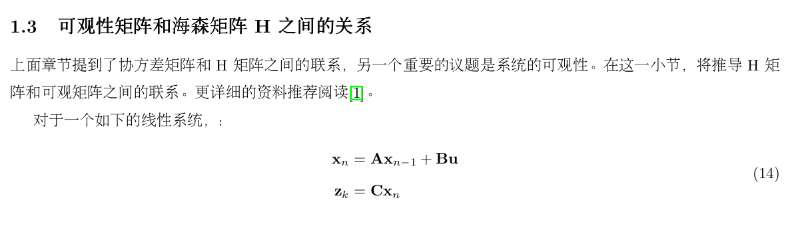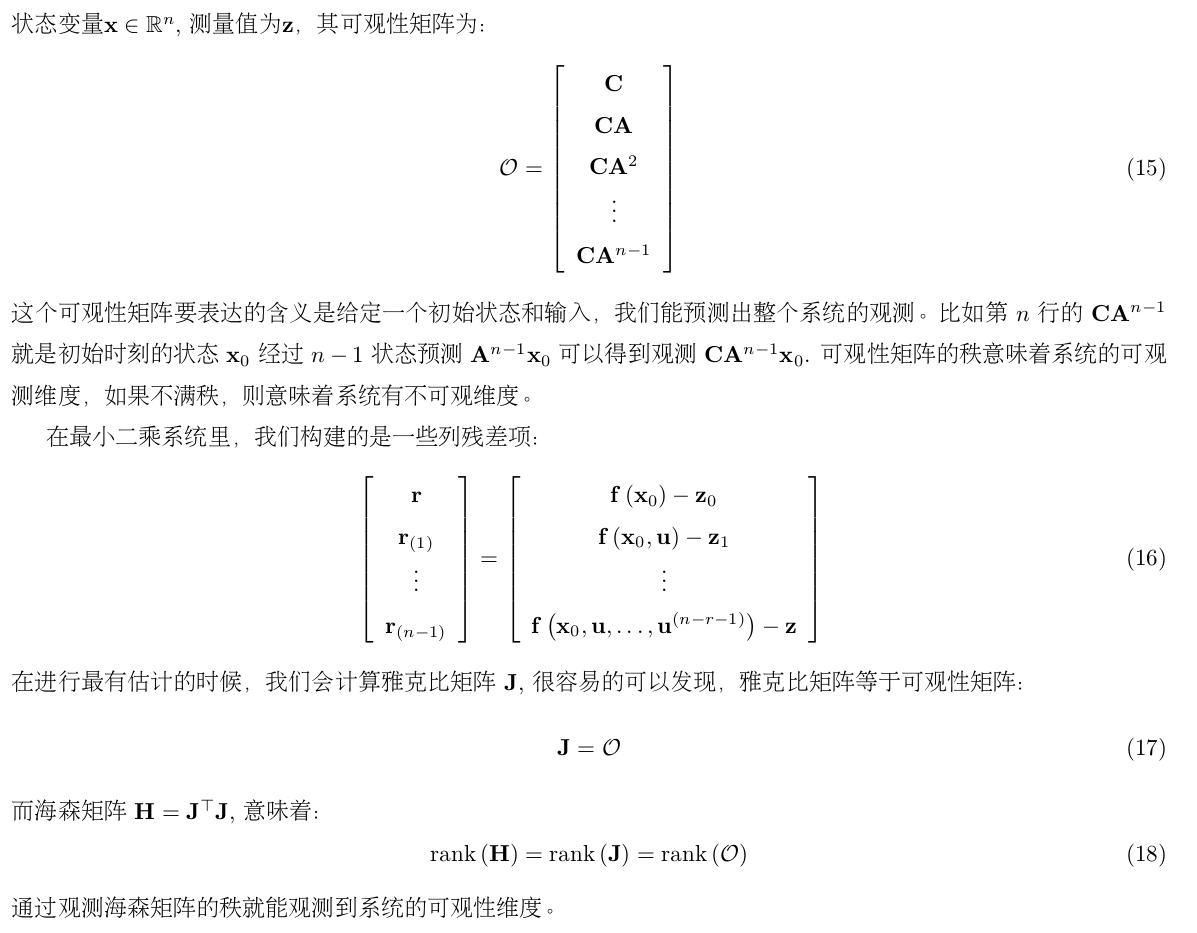## NEES (normalized estimation error squared)

• NEES closer to 6 for VIO

# Inconsistency of Estimator

a state estimator is consistent if the estimation errors (i) are zero-mean, and (ii) have covariance matrix smaller or equal to the one calculated by the filter.

## Degeneracy (Insufficient Restraint) / Inconsistency in SLAM

### Motion

• constant acceleration
• pure translation

## Maintain(Solve) Consistency(Inconsistency)

paper:

• VINS on Wheels
• Odometry measurements
• Planar-motion constraints

### FEJ (First-Estimate Jacobians)

• paper: A First-Estimates Jacobian EKF for Improving SLAM Consistency

• estimation from the first time

to ensure that the state transition and Jacobian matrices are evaluated at correct linearization points such that the above observability analysis will hold true

FEJ 算法：不同残差对同一个状态求雅克比时，线性化点必须一致，这样就能避免零空间退化而使得不可观变量变得可观。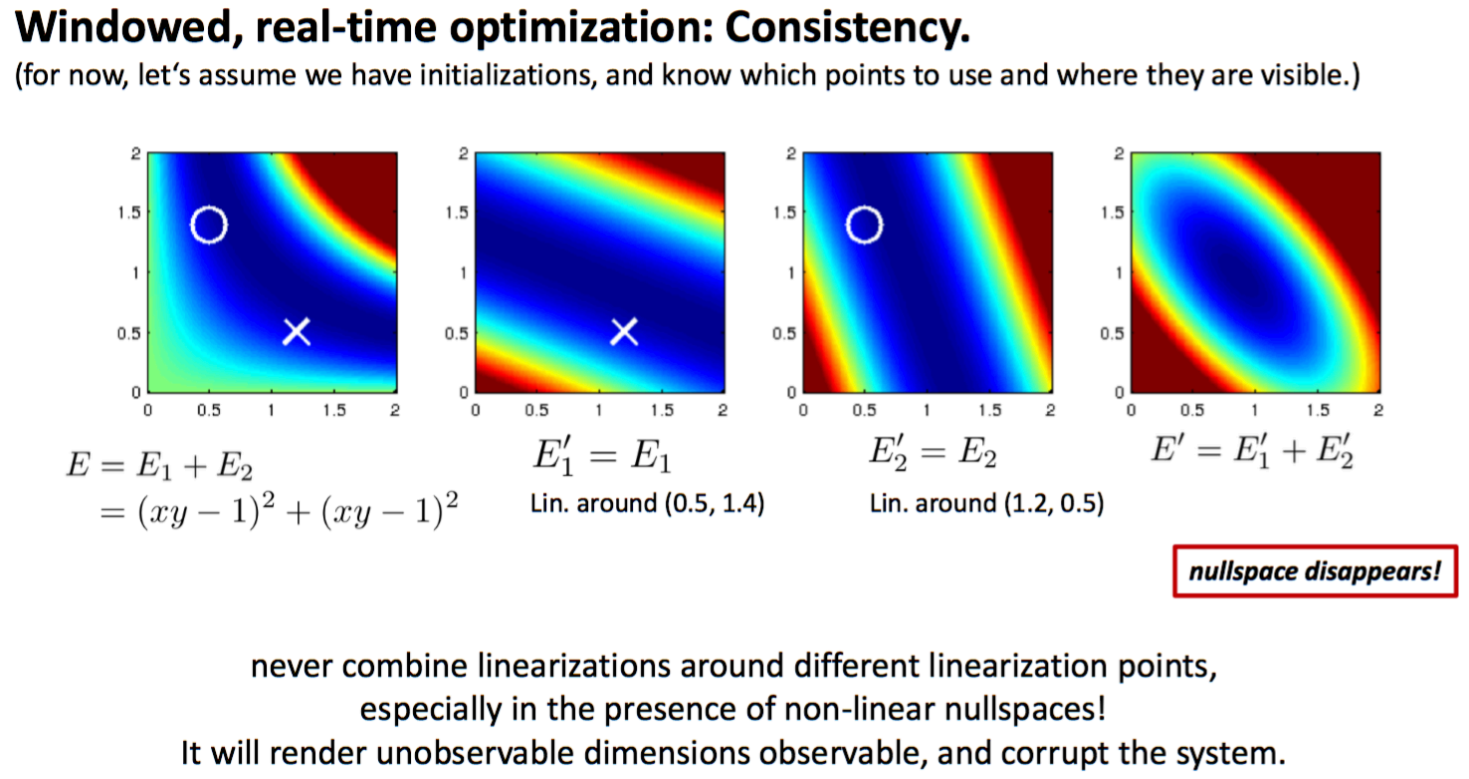app:

• OKVIS
• DSO
• ElasticFusion 改进版 (IROS2107, Stefan Leutenegger)
• OpenVINS

ref:

TODO

### Observability Constraint (OC)-VINS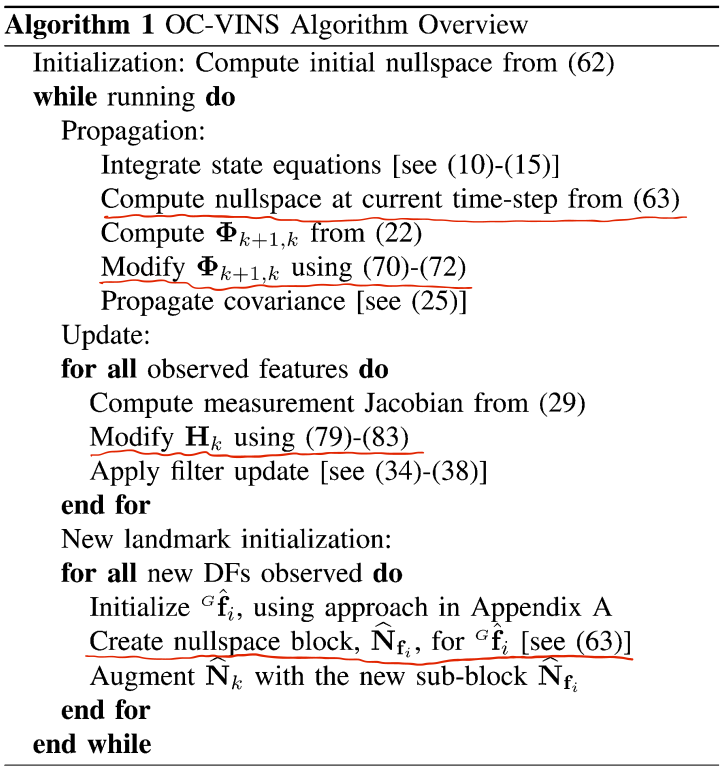#### App: OC-MSC-KF

MSCKF-VIO (S-MSCKF):

Modification of the State Transition Matrix $$\Phi$$

Modification of the Measurement Jacobian $$H$$

# Gauge Freedom Handling

It is well known that visual-inertial systems have four degrees of freedom that are not observable: the global position and the rotation around gravity. These unobservable degrees of freedom (called gauge freedom) have to be handled properly in visual-inertial state estimation to obtain a unique state estimate.

H有正确的零空间，比如，对于单目VO，rank(N(H)) = 7，则H为奇异矩阵，那么增量方程始终存在病态或求解不稳定问题；通过处理 规范自由度 解决。

In optimization-based methods, three approaches are usually used:

• Gauge Fixation: fixing the initial state,
• Gauge Prior: adding a prior to the initial state,
• Free Gauge: allowing the parameters to evolve freely during optimization.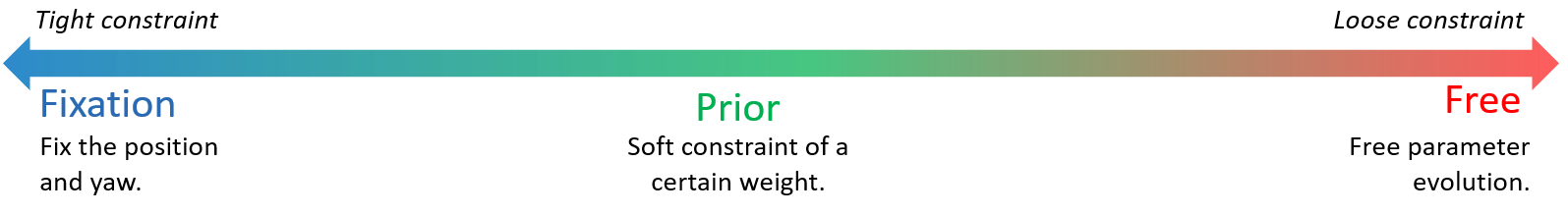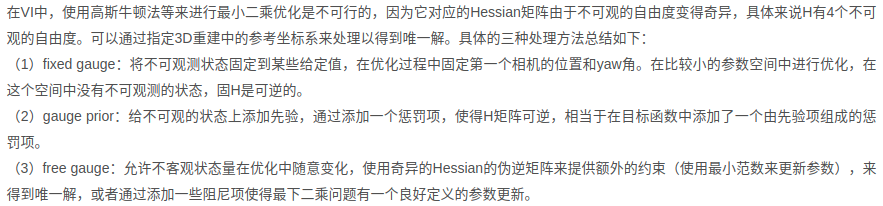ref:

# FAQ

• 为什么位置不可观，对于单目VO，第一帧不是固定住了吗？

# Reference

Observability and Inconsistency in a Nutshell
https://cgabc.xyz/posts/881febbe/

Gavin Gao

2022年7月16日